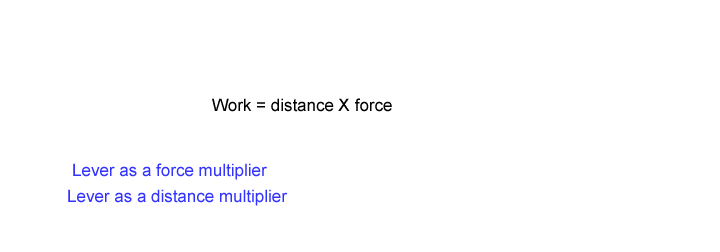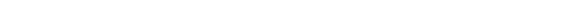Look at the simplified animations above. Click on the "Lever as a force multiplier" What do you notice happens when the effort is closer to the fulcrum than the load? Can a small amount of effort lift a larger load? In other words can I magnify a force using a lever? What is the trade-off when we move the effort closer to the fulcrum? Now work is a term we are all familiar with. We know that when we do work we generally apply some force. Assume you are pushing a heavy wheel barrow. Obviously you are doing work. But are you doing more work when you push the wheelbarrow 100m as opposed to just 10m? Definitely you are. It is obvious in real life that more work is done if a force is applied over a longer distance. So we define work as: Work = force X distance Look at the simplified animation once more. We do work on one side of the lever when we push down on it and the lever does some work on the load pushing it up. Knowing the formula for work, what can you deduce about the work done on both sides of the lever? If I convert a lever to a distance multiplier, where must the effort and load be relative to the fulcrum? A lever acting as a distance mulitplier is also known as a speed multiplier. Explain.